• 选择排序思想跟插入排序很像，也将数组分为未排序区间和已排序区间。不同的是，选择排序是将未排序区间中选择一个最小值放在已排序区间的后边。跟插入排序不同，不需要不停往后移动数据，选择排序直接替换最小值到...
选择排序思想跟插入排序很像，也将数组分为未排序区间和已排序区间。不同的是，选择排序是将未排序区间中选择一个最小值放在已排序区间的后边。跟插入排序不同，不需要不停往后移动数据，选择排序直接替换最小值到指定位置即可。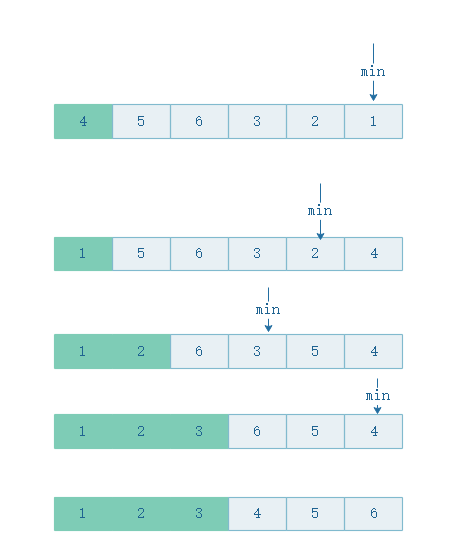代码也很简单：

 /**
* 选择排序
* @param a
* @param n
*/
public static void selectionSort(int[] a, int n) {
if (n <= 1) {
return;
}

for (int i = 0; i < n - 1; ++i) {
// 查找最小值
int minIndex = i;
for (int j = i + 1; j < n; ++j) {
if (a[j] < a[minIndex]) {
minIndex = j;
}
}

// 交换
int tmp = a[i];
a[i] = a[minIndex];
a[minIndex] = tmp;
}
}

选择排序是原地排序算法吗？

选择排序不需要额外的存储空间，空间复杂度为O(1),所以是原地排序算法。

选择排序是稳定排序算法吗？

不稳定，比如[5,8,5,2,9]这个数组，使用选择排序算法第一次找到的最小元素就是2，与第一个位置的元素5交换位置，那第一个5和中间的5的顺序就变量，所以就不稳定了。所以选择排序算法 比起插入排序和冒泡排序还是稍微逊色。

选择排序算法的时间复杂度是多少？

最好、最差、平均时间复杂度都是O(n^2),因为无论你是否完全有序，还是完全逆序，都需要找出后边的最小值进行替换。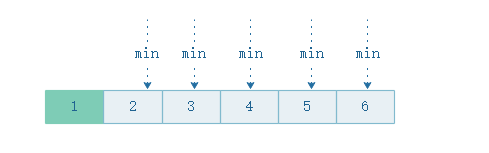展开全文算法 数组
• 排序算法之 冒泡排序及性能优化（时间复杂度+空间复杂度分析） 简单选择排序 基本思想：比较+交换 1. 从待排序序列中，找到关键字最小的元素； 2. 如果最小元素不是待排序序列的第一个元素，将其和第一个元素互换；...

排序算法之 冒泡排序及性能优化（时间复杂度+空间复杂度分析）

简单选择排序
基本思想：比较+交换
1. 从待排序序列中，找到关键字最小的元素；
2. 如果最小元素不是待排序序列的第一个元素，将其和第一个元素互换；
3. 从余下的 N - 1 个元素中，找出关键字最小的元素，重复(1)、(2)步，直到排序结束。
因此我们可以发现，简单选择排序也是通过两层循环实现。
第一层循环：依次遍历序列当中的每一个元素
第二层循环：将遍历得到的当前元素依次与余下的元素进行比较，符合最小元素的条件，则交换。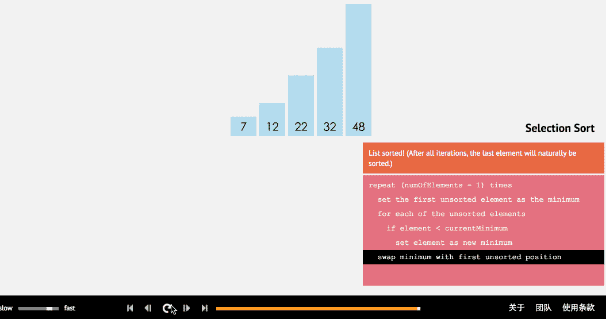排序算法实现
int i,j,k，temp;
for(i=0; i<n-1; i++) //依次遍历序列中每一个元素，进行n-1轮，最后一轮只剩下最后一个元素
{
k = i; //默认该轮的第一个元素是最小值
for(j=i+1; j<n; j++) //遍历其余元素
{
if(arr[j] < arr[k]) //若其余元素有比该轮第一个元素小的，则记录元素下标
k = j; //记录元素下标
}
if(k != i) //进行交换
{
temp = arr[k];
arr[k] = arr[i];
arr[i] = temp;
}
}

简单选择排序时间复杂度分析
此排序的最大特点就是交换移动数据次数相当少，节约了相应的时间
无论最好还是最坏情况，其比较次数都是一样多的，共需要比较 （n-1）+ （n-2）+…+ 2 + 1 = n * (n-1)/2次；
而对交换次数而言，当最好的时候，交换0次，最差时，交换n-1次;
基于最终的排序时间是比较和交换次数总和，因此，总的时间复杂度依然为O(n^2)，但性能优于冒泡排序。


展开全文算法 数据结构
• # include <stdio.h> void print(int * a, int len) { for(int i=0; i<len; i++) printf("%d ", a[i]); printf("\n"); } void swap(int * a, int * b) { int t = *a; *a = *b;... i+
# include <stdio.h>

void print(int * a, int len)
{
for(int i=0; i<len; i++)

printf("%d ", a[i]);
printf("\n");

}

void swap(int * a, int * b)
{
int t = *a;
*a = *b;
*b = t;
}

void selsort(int * a, int len)
{

for(int i=0; i<len; i++)//执行n次
{
int min=i;

for(int j=i+1; j<len; j++)//执行n-1加到1次，近似于n的平方次
if(a[j]<a[min])
min = j;

swap(&a[i], &a[min]);
}

}

int main(void)
{
int a = {8, 5, 6, 1, 5};

selsort(a, 5);

print(a, 5);

return 0;
}



展开全文• 排序算法之 简单选择排序时间复杂度分析 排序算法之 直接插入排序及时间复杂度分析 排序算法之 希尔排序及时间复杂度分析 排序算法之 快速排序及时间复杂度分析 排序算法之 堆排序及时间复杂度分析 归并排序 归并...

排序算法之 冒泡排序及性能优化（时间复杂度+空间复杂度分析）
排序算法之 简单选择排序及时间复杂度分析
排序算法之 直接插入排序及时间复杂度分析
排序算法之 希尔排序及时间复杂度分析
排序算法之 快速排序及时间复杂度分析
排序算法之 堆排序及时间复杂度分析

归并排序
归并排序是建立在归并操作上的一种有效排序算法，该算法是采用分治法的一个典型应用。
这里的分治如何理解？
比如我们要统计本县城的高考状元，而一个县城中有很多中学，每个中学又有若干个班级，每个班级有若干名学生，每个学生是一个单独个体，看成数组中的一个元素。接下来，班级内学生两两组合并排好序组成一组，然后两组两组再进行合并并排好序…各班级分别排好序…各学校分别排好序…县中所有学生排序…
归并排序基本思想：

将序列中待排序数分为若干组，每个数字为一组
将若干个组进行两两合并，保证合并后的组是有序的
一直重复第二步操作直到剩下一组，排序完成

效果图：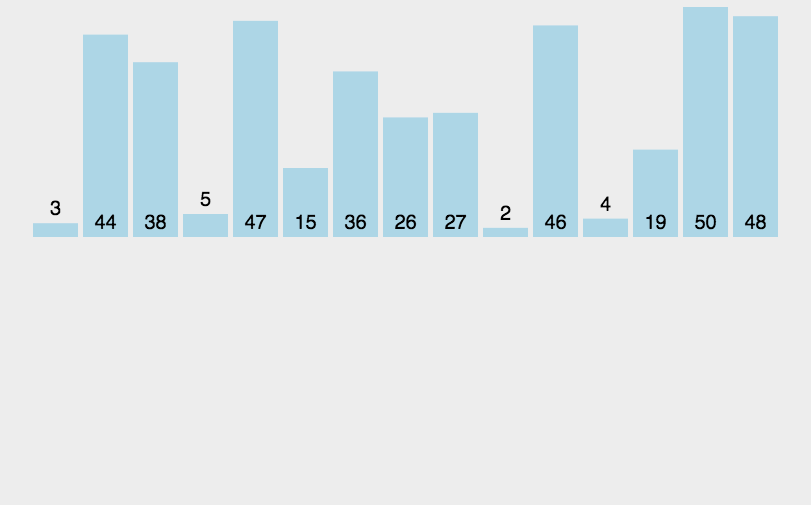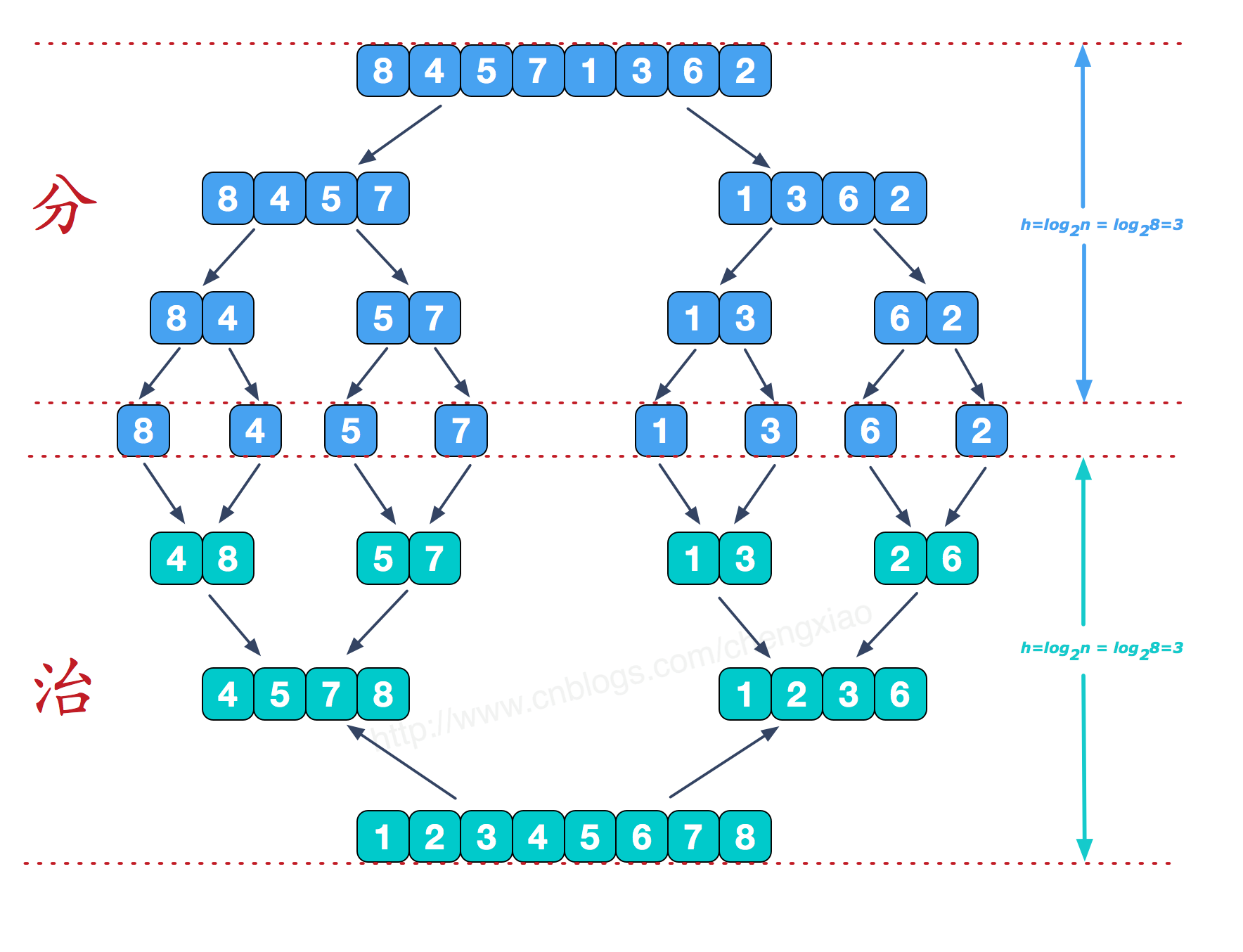算法实现
#include <stdio.h>

int arr1 = {9,8,7,6,5,4,3,2,1,0}, arr2;//原数组arr1，临时空间数组arr2
void merge(int low, int mid, int high) {
int i = low, j = mid + 1, k = low;
while (i <= mid && j <= high)
if (arr1[i]<arr1[j])
arr2[k++] = arr1[i++];
else
arr2[k++] = arr1[j++];
while (i <= mid)
arr2[k++] = arr1[i++];
while (j <= high)
arr2[k++] = arr1[j++];
for (i = low; i <= high; i++) {
arr1[i] = arr2[i];
}
}

void mergeSort(int a, int b) {
//直到a=b时，停止递归。
if (a<b) {
int mid = (a + b) / 2;
mergeSort(a, mid);
mergeSort(mid + 1, b);
merge(a, mid, b);
}
}

int main() {
int i;
mergeSort(0, 9);
return 0;
}


时间复杂度
归并排序的效率是比较高的，设数列长为N，将数列分开成小数列一共要logN步，每步都是一个合并有序数列的过程，时间复杂度可以记为O(N)，故一共为O(NlogN)。因为归并排序每次都是在相邻的数据中进行操作，所以归并排序在O(NlogN)的几种排序方法（快速排序，归并排序，希尔排序，堆排序）也是效率比较高的。


展开全文算法 数据结构
• 选择排序是不稳定的排序方法。 选择排序是给每一个位置选择当前元素最小的，比方给第一个位置选择最小的，在剩余元素里面给第二个元素选择第二小的，依次类推。直到第n-1个元素，第n个元素不用选择了，由于仅仅剩下...
• 从当前未排序的整数中找到最小的整数，将它放在已排序的整数列表的最后。 #include&lt;iostream&gt; #include&lt;Windows.h&gt; #include&lt;time.h&gt; using namespace std; void Select...数据结构
• 排序算法之 简单选择排序时间复杂度分析 排序算法之 直接插入排序及时间复杂度分析 排序算法之 希尔排序及时间复杂度分析 排序算法之 快速排序及时间复杂度分析 堆排序 堆的概念： 本质是一种数组对象。特别重要...算法 数据结构
• 排序算法之 简单选择排序时间复杂度分析 排序算法之 直接插入排序及时间复杂度分析 排序算法之 希尔排序及时间复杂度分析 快速排序 科普： 快速排序算法最早由图领奖获得者Tony Hoare设计出来的，是上世界最伟大...算法
• 排序算法之 简单选择排序时间复杂度分析 排序算法之 直接插入排序及时间复杂度分析 希尔排序 算法思想：将整个待排序列分割成若干个子序列（由相隔增量个元素组成），分别进行直接插入排序，然后依次缩小增量再...算法 数据结构
• //选择排序时间复杂度分析：最好最坏情况最内层的循环平均执行N/2次（必须比较j和min处元素的值），外层循环执行N次，所以复杂度O(N^2) 下面说一说时间复杂度的分析方法： 这里把算法分为分治和非分治两种情况...
• 认识时间复杂度 常数时间的操作：一个操作如果和数据量没有关系，每次都是固定时间内完成的操作，叫做常数操作。 时间复杂度为一个算法...评价一个算法流程的好坏，先看时间复杂度的指标，然后再分析不同数据样本下...
• 排序算法之 简单选择排序时间复杂度分析 直接插入排序 直接插入排序的核心思想就是：将数组中的所有元素依次跟前面已经排好的元素相比较，如果选择的元素比已排序的元素小，则交换，直到全部元素都比较过。 因此...算法 数据结构
• 时间复杂度(平均) 最差情况 最好情况 空间复杂度 冒泡排序 O(n2) O(n2) O(n) O(1) 选择排序 O(n2) O(n2) O(n2) O(1) 插入排序 O(n2) O(n2) O(n) O(1) 快速排序 O(nlogn) O(n2) O(nlogn) O...
• 之前一直用Java实现代码，最近开始入门Python，同时复习下简单的算法，以下尝试用Python实现选择排序，并分析时间复杂度。 代码实现： def select_search(list): l = len(list) for i in range (0,l): ...python 算法
• 24功能之各种排序时间复杂度分析 1 稳定的排序 1）冒泡 2）插入 3）归并 2 不稳定的排序 1)选择排序 2)希尔排序 3）快速排序 参考快排分析 4）堆排序 参考添加链接描述 3 最后给出各种排序O(N)的总结表与排序...数据结构 算法
• 排序法最差时间分析平均时间复杂度稳定度空间复杂度冒泡排序O(n2)O(n2)稳定O(1)快速排序O(n2)O(n*log2n)不稳定O(log2n)~O(n)选择排序O(n2)O(n2)稳定O(1)二叉树排序O(n2)O(n*log2n)不一顶O(n)插入排序O(n2)O(n2)稳定O...
• 排序(Sorting) 是计算机程序设计中的一种重要操作，它的功能是将一个数据元素（或记录）的...本文主要介绍快速排序算法和归并排序算法的基本概念、原理以及具体的实现方法，并对这两种排序算法的时间复杂度进行分析
• 选择排序（Selection sort）是一种简单直观的排序算法。它的工作原理是：第一次从待排序的数据元素中选出最小（或最大）的一个元素，存放在序列的起始位置，然后再从剩余的未排序元素中寻找到最小（大）元素，然后放...
• 快速排序的思想是在数组[p,r]中选择一个分区点q，将数组一分为2，同时将小于分区点的数值的放到分区点左侧[p,q-1]，大于分区点的数值的放到分区点右侧[q+1,r],重复这个过程。 快速排序也是用到了分治思想和递归实现...算法
• 蛮力法  蛮力法又称穷举法和枚举法,是一种简单直接地解决问题的方法,常常直接基于问题的描述,所以蛮力法也是最容易应用的方法。... 常用的蛮力法应用算法有我们比较熟悉的冒泡排序、选择排序。 选择排...
• 快速排序思想： 1.选择数组左边第一个元素为枢轴pivot，把数组所有元素比pivot大的放在...平均时间复杂度分析： T(1) = 1; T(n) = 2*T(n/2) + a*n;(a为常数，每次合并时，复杂度为O(n)) = 2*(2*T(n/4)+a*n/2) + a*
• 选择排序原理：从N个未排序的数据项中选出最小数（这里假设我们按照升序排列），再从剩下的N-1个未排序的数据项中选出最小数，不断重复此过程，直到所有数被拍好序为止。 以下为实现代码： #!/usr/bin/python ...python Python 插入排序
• 1、插入排序 最佳情况：O(n) 最差情况：O(nlgn) 平均情况：O(nlgn)2、选择排序 在所有情况下的时间复杂度均为O(n*n)3、冒泡排序 最佳情况：O(n) 最差情况：O(n*n) 平均情况：O(n*n)4、归并排序 所有情况下的...
• 生成伪随机序列，用选择排序法测试排序时间，系统输出排序时间，多次测试，记录结果验证选择排序算法的时间负责度。...